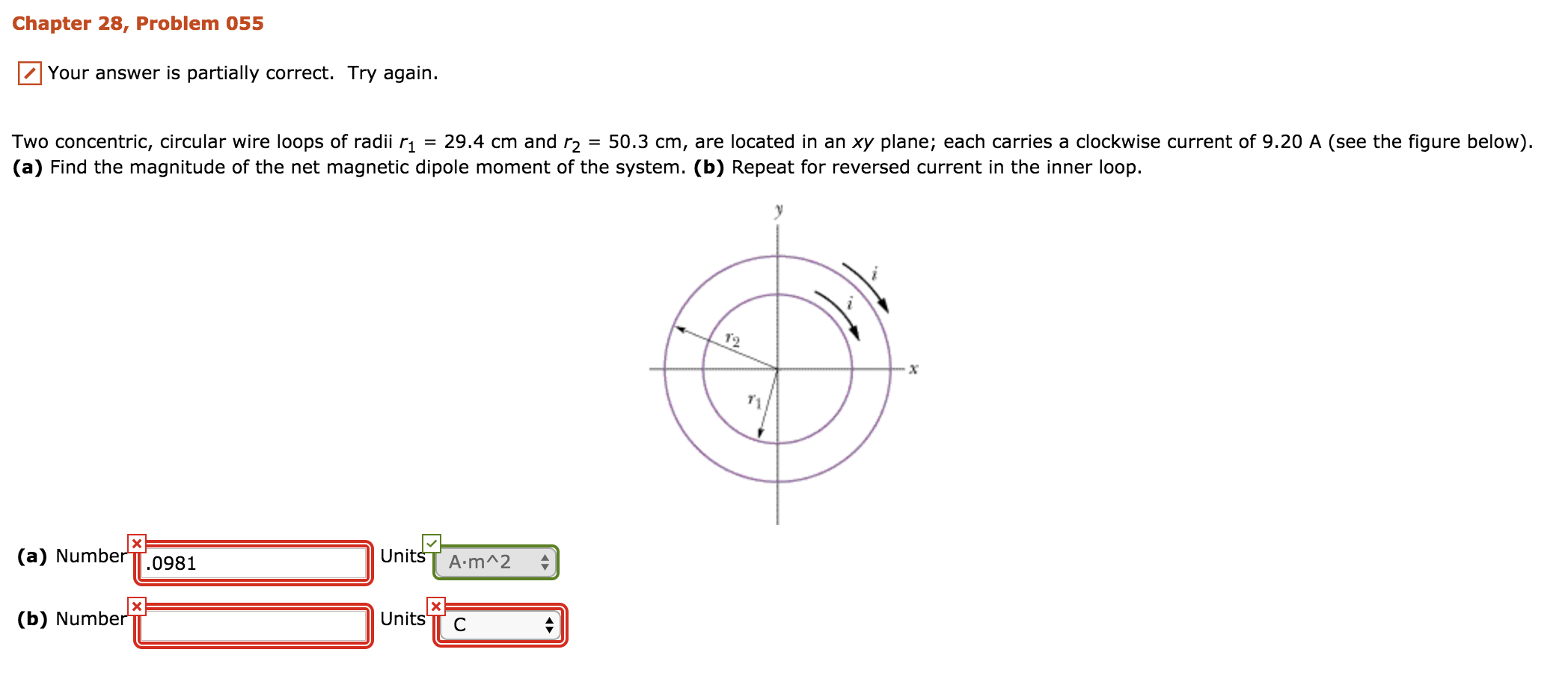# Chapter 28, Problem 055Your answer is partially correct. Try again.Two concentric, circular wire loops of radii r1 = 29.4 cm and r2 = 50.3 cm, are located in an xy plane; each carries a clockwise current of 9.20 A (see the figure below)(a) Find the magnitude of the net magnetic dipole moment of the system. (b) Repeat for reversed current in the inner loopUnitss A.mA2(a) Number TT.0981Units TT C(b) Number

Question
468 viewshelp_outlineImage TranscriptioncloseChapter 28, Problem 055 Your answer is partially correct. Try again. Two concentric, circular wire loops of radii r1 = 29.4 cm and r2 = 50.3 cm, are located in an xy plane; each carries a clockwise current of 9.20 A (see the figure below) (a) Find the magnitude of the net magnetic dipole moment of the system. (b) Repeat for reversed current in the inner loop Unitss A.mA2 (a) Number TT.0981 Units TT C (b) Number fullscreen
check_circle

Step 1

Given:

Radius of inner loop, r1= 29.4 cm = 0.294 m

Radius of outer loop, r= 50.3 cm = 0.503 m

Current in each loop, i = 9.20 A

We need to find the net magnetic dipole moment of the system.

Step 2

Magnetic dipole moment can be calculated.

The direction of magnetic dipole moment is the direction of the magnetic field generated by the current carrying loop which is given by Right-hand thumb rule.

Step 3

The net diplole moment of the system is: 9.80 A.m2.

The two dipole moments have b...

### Want to see the full answer?

See Solution

#### Want to see this answer and more?

Solutions are written by subject experts who are available 24/7. Questions are typically answered within 1 hour.*

See Solution
*Response times may vary by subject and question.
Tagged in

### Physics